Tools/Reasoning sub-menu provide a wizard to sum and normalize facts of the project: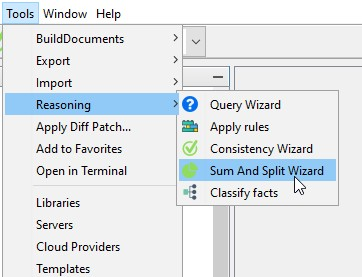Sum

Knowledge base is organized by sets of facts.Sometime it can be interesting to sum these graphs. Example below presents two graphs first contains geographical information,second contains information about flavor and sweetness.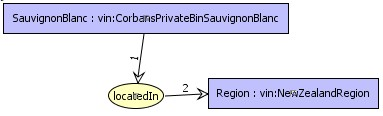neither graph1 nor graph2 match with this query: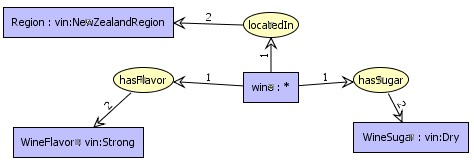CoGui provides a wizard to sum graphs. It consist in merging graphs into the same sum graph.
the resulting graph is shown below :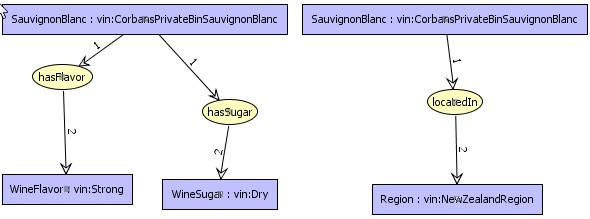not normalized sum of graph1 and graph2

Normalize

We obtain a sum graph which is not a normal simple graph. A normal SG is such that each concept is uniquely coreferent with itself. Note that an implicit coreference link exists between the two nodes representing the individual vin:CorbansPrivateBinSauvignonBlanc. Normalization performs the fusion of nodes associated to the same individual. See below the normalized sum of graph1 and graph2. Resulting graph give an answer to query above.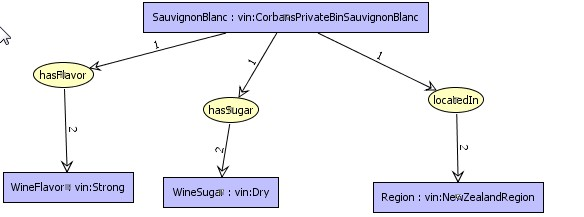normalized sum of graph1 and graph2

Sum and Split wizard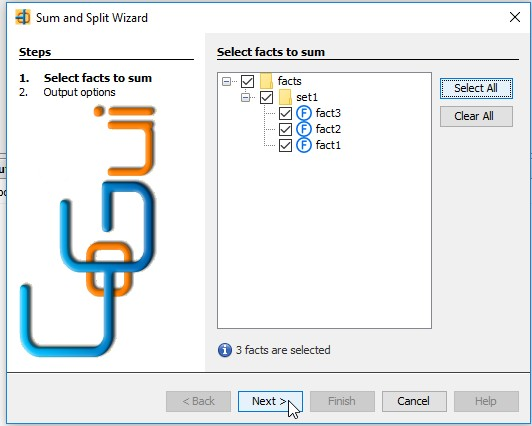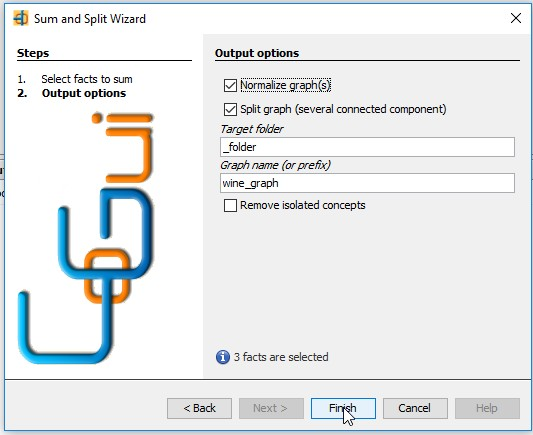Select facts After the sum has been computed, the graph will be normalized and split when there is several connected components

Created with the Personal Edition of HelpNDoc: Easily create Web Help sites